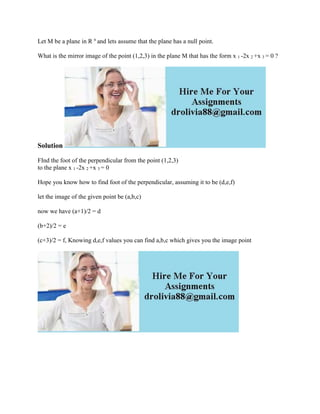Diese Präsentation wurde erfolgreich gemeldet.

# Let M be a plane in Rn and lets assume that the plane has a null point.docx

Anzeige
Anzeige
Anzeige
Anzeige
Anzeige
Anzeige
Anzeige
Anzeige
Anzeige
Anzeige
Anzeige×

1 von 1 Anzeige

# Let M be a plane in Rn and lets assume that the plane has a null point.docx

Let M be a plane in R n and lets assume that the plane has a null point.
What is the mirror image of the point (1,2,3) in the plane M that has the form x 1 -2x 2 +x 3 = 0 ?
Solution
FInd the foot of the perpendicular from the point (1,2,3)
to the plane x 1 -2x 2 +x 3 = 0
Hope you know how to find foot of the perpendicular, assuming it to be (d,e,f)
let the image of the given point be (a,b,c)
now we have (a+1)/2 = d
(b+2)/2 = e
(c+3)/2 = f, Knowing d,e,f values you can find a,b,c which gives you the image point
.

Let M be a plane in R n and lets assume that the plane has a null point.
What is the mirror image of the point (1,2,3) in the plane M that has the form x 1 -2x 2 +x 3 = 0 ?
Solution
FInd the foot of the perpendicular from the point (1,2,3)
to the plane x 1 -2x 2 +x 3 = 0
Hope you know how to find foot of the perpendicular, assuming it to be (d,e,f)
let the image of the given point be (a,b,c)
now we have (a+1)/2 = d
(b+2)/2 = e
(c+3)/2 = f, Knowing d,e,f values you can find a,b,c which gives you the image point
.

Anzeige
Anzeige

### Let M be a plane in Rn and lets assume that the plane has a null point.docx

1. 1. Let M be a plane in R n and lets assume that the plane has a null point. What is the mirror image of the point (1,2,3) in the plane M that has the form x 1 -2x 2 +x 3 = 0 ? Solution FInd the foot of the perpendicular from the point (1,2,3) to the plane x 1 -2x 2 +x 3 = 0 Hope you know how to find foot of the perpendicular, assuming it to be (d,e,f) let the image of the given point be (a,b,c) now we have (a+1)/2 = d (b+2)/2 = e (c+3)/2 = f, Knowing d,e,f values you can find a,b,c which gives you the image point